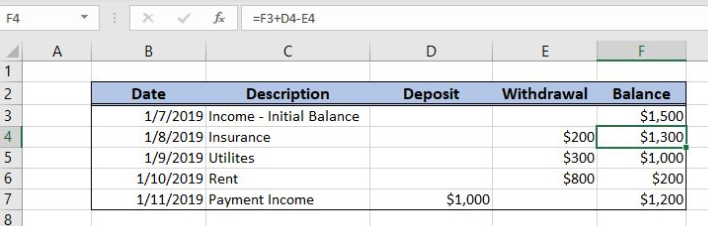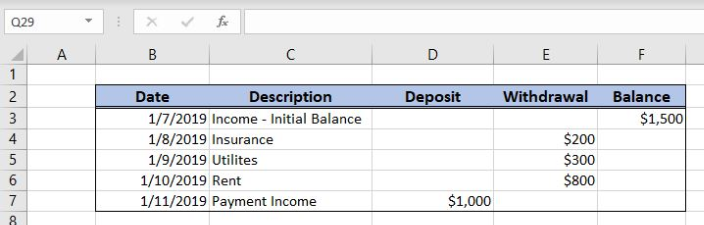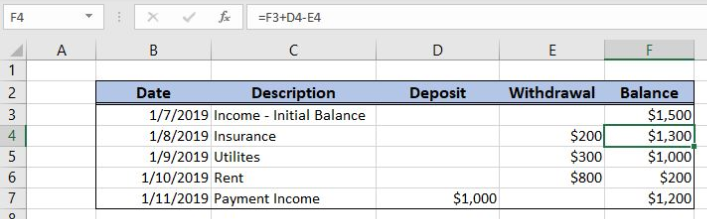Get instant live expert help with Excel or Google Sheets“My Excelchat expert helped me in less than 20 minutes, saving me what would have been 5 hours of work!”

#### Post your problem and you’ll get Expert help in seconds.

Your message must be at least 40 characters
Our professional Expert are available now. Your privacy is guaranteed.

# Check register balance

Excel allows a user to calculate a check register balance using the simple formula. Current balance is calculated when all deposits are summed and all withdrawals are subtracted. This step by step tutorial will assist all levels of Excel users in calculating a check register balance.Figure 1. The result of the formula

## Syntax of the Formula

The generic formula is:

`=previous_balance + deposit - withdrawal`

The parameters of the formula are:

• previous_balance – a balance from the previous period
• deposit – a cash income
• withdrawal – a cash withdrawal.

## Setting up Our Data for the Example

Let’s look at the structure of the data we will use. In column B, we have dates, column C we have descriptions of deposit or withdrawal. In column D, there are deposits, while in column D, we have withdrawals. Finally, in column E, we want to get a current balance.Figure 2. Data that we will use in the example

## Calculating the Check Register Balance

In our example, we want to get the current balance for 1/8/2019, in the cell F4. Therefore, we must take in count the previous balance from F3 and deposit and withdrawal from D4 and E4.

The formula looks like:

`=(B3 - C3)/B3`

The parameter previous_balance is the cell F3. The cell D4 is the deposit, while E4 is the withdrawal.

To apply the formula, we need to follow these steps:

• Select cell F4 and click on it
• Insert the formula: `=(B3 - C3)/B3`
• Press enter
• Drag the formula down to the other cells in the column by clicking and dragging the little “+” icon at the bottom-right of the cell.Figure 3. Calculating the check register balance

The previous balance is \$1,500, there are no deposits for 1/8/2019 and the withdrawal is \$200. Therefore, the balance is \$1,300 in the cell F4.

Most of the time, the problem you will need to solve will be more complex than a simple application of a formula or function. If you want to save hours of research and frustration, try our live Excelchat service! Our Excel Experts are available 24/7 to answer any Excel question you may have. We guarantee a connection within 30 seconds and a customized solution within 20 minutes.

### Did this post not answer your question? Get a solution from connecting with the expert.Another blog reader asked this question today on Excelchat:## Subscribe to Excelchat.coAnother blog reader asked this question today on Excelchat: